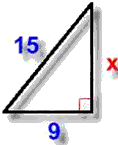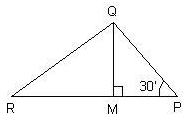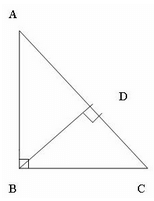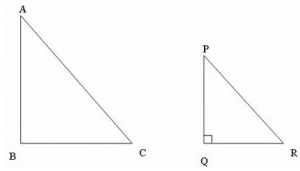Test: Pythagoras Theorem

# Test: Pythagoras Theorem - Class 10

Test Description

## 15 Questions MCQ Test Online MCQ Tests for Class 10 - Test: Pythagoras Theorem

Test: Pythagoras Theorem for Class 10 2023 is part of Online MCQ Tests for Class 10 preparation. The Test: Pythagoras Theorem questions and answers have been prepared according to the Class 10 exam syllabus.The Test: Pythagoras Theorem MCQs are made for Class 10 2023 Exam. Find important definitions, questions, notes, meanings, examples, exercises, MCQs and online tests for Test: Pythagoras Theorem below.
Solutions of Test: Pythagoras Theorem questions in English are available as part of our Online MCQ Tests for Class 10 for Class 10 & Test: Pythagoras Theorem solutions in Hindi for Online MCQ Tests for Class 10 course. Download more important topics, notes, lectures and mock test series for Class 10 Exam by signing up for free. Attempt Test: Pythagoras Theorem | 15 questions in 15 minutes | Mock test for Class 10 preparation | Free important questions MCQ to study Online MCQ Tests for Class 10 for Class 10 Exam | Download free PDF with solutions
 1 Crore+ students have signed up on EduRev. Have you?
Test: Pythagoras Theorem - Question 1

### Which of the following cannot be the sides a right triangle?​

Detailed Solution for Test: Pythagoras Theorem - Question 1

H= P+ B2
H2 = 81
P+ B= 25 + 49 = 74
H ≠ P2+B2.Therefore the sides cannot form a right triangle.

Test: Pythagoras Theorem - Question 2

### In a triangle ABC, AC= √180, AB = 6, BC = 12. What is ∠B = ?

Detailed Solution for Test: Pythagoras Theorem - Question 2

We have AB2=36 and BC2=144
Adding both the squares we get
AB2+BC2=36+144=180
=AC2
So , AC is the hypotenuse according to pythagoras theorem , So the angle opposite to it which is B is 90 degrees.

Test: Pythagoras Theorem - Question 3

### From the given figure, find the unknown x.​

Detailed Solution for Test: Pythagoras Theorem - Question 3

Since the triangle is a right angled triangle,
Applying Pythagoras Theorem,
H2=P2+B2
152=P2+92
P2=225-81=144
P=12

Test: Pythagoras Theorem - Question 4

Three squares are based on the sides of a right angled triangle. The area of the two smaller ones are 144 sq. cm and 256 sq. cm. What is the area of the third one?​

Test: Pythagoras Theorem - Question 5

What is the diagonal length of a TV screen whose dimensions are 80 x 60 cm?

Detailed Solution for Test: Pythagoras Theorem - Question 5

We have a TV which is a rectangle whose adjacent sides form an angle of 90 degrees so The triangle formed by the two sides and a diagonal will be a right angle. So, Applying Pythagoras Theorem,
(Diagonal)2=802+602=6400+3600=10000
Diagonal=100cm

Test: Pythagoras Theorem - Question 6

Which of the following is a Pythagorean triplet ?​

Detailed Solution for Test: Pythagoras Theorem - Question 6

A Pythagorean triple consists of three positive integers a, b, and c, such that a² + b² = c².

a) 432 = 1849

362+182 = 1620

b) 252  = 625

15+ 202 =625

c) 132 = 169

32 + 122 = 153

d) 262 = 676

242 + 252 = 1201

so option b is correct

Test: Pythagoras Theorem - Question 7

QM ⊥ RP and PR2 – PQ2 = OR2. If∠QPM = 30°, Then ∠MQR isDetailed Solution for Test: Pythagoras Theorem - Question 7

In triangle PMQ
Angle M = 90°
Angle P = 30°
Angle QMP + Angle MPQ + Angle PQM = 180 degree ( By angle sum property )
90 + 30 + Angle PQM = 180
Angle PQM = 180 - 120
Angle PWM = 60 Degree
QM divides Angle PQR into two equal parts .
Therefore Angle MQR = 30°

Test: Pythagoras Theorem - Question 8

In the above figure, AB = c, BC = a, AC = b, AD = y, DB = p. Check which of the following options is correct ?​Test: Pythagoras Theorem - Question 9

In right triangled ABC right angled at B, a line DE is drawn through the mid point D of AB and parallel to BC. If AB = 9 cm, BC = 12 cm. AE = ?​

Detailed Solution for Test: Pythagoras Theorem - Question 9

In a triangle ABC in which angle B = 90 degree
So, AB is height of the given triangle = 9 cm
Base of triangle i.e, BC = 12 cm
DE is || to BC
D is the mid point of side AB and E is the mid point of side AC
By applying pythagoras
AC2 = AB2 + BC2
AC2 = 9^2 + 12^2
AC2 = 81 + 144
AC2 = 225
AC = root under 225
AC = 15 cm
E is the mid point of AC
Therefore AE = AC / 2
AE = 15 / 2
AE = 7.5 cm

Test: Pythagoras Theorem - Question 10

A boy is trying to catch fish sitting at a height of 12 m from the surface of the water. A big fish is at a horizontal distance of 5 m from him. What should be the length of his string to get the fish?

Test: Pythagoras Theorem - Question 11

Three numbers form a Pythagorean triplet. Two of them are 15 and 17 where 17 is the largest of them. The third number is

Test: Pythagoras Theorem - Question 12

Triangle PQR is an isosceles right triangle right angled at Q. If PR = √50. What is the value of each of the equal sides?​

Test: Pythagoras Theorem - Question 13

The length of an altitude of an equilateral triangle of side a is:

Test: Pythagoras Theorem - Question 14

Two friends A and B start from the same point in the Eastern and Northern directions at the same time. How far are they from each other when A has travelled 5 km and B has travelled 12 km. distance?​

Test: Pythagoras Theorem - Question 15

Two similar right triangles ABC and PQR are as shown in the figure.If AB = √3 , PQ = √3/2,BC = 1. Find PR = ?## Online MCQ Tests for Class 10

615 tests
Information about Test: Pythagoras Theorem Page
In this test you can find the Exam questions for Test: Pythagoras Theorem solved & explained in the simplest way possible. Besides giving Questions and answers for Test: Pythagoras Theorem, EduRev gives you an ample number of Online tests for practice

615 tests